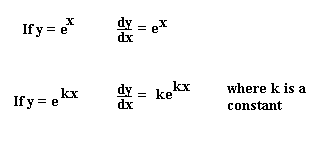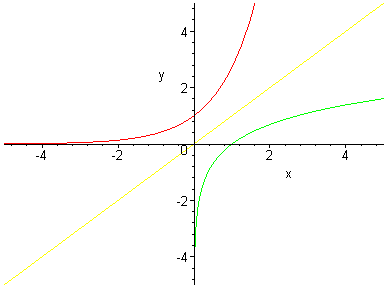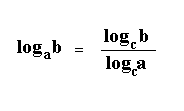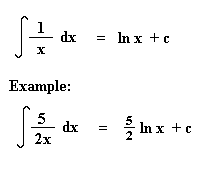## Exponentials and Logarithms

The Exponential Function

The exponential function, written exp(x) or ex, is the function whose derivative is equal to its equation. In other words:Because of this special property, the exponential function is very important in mathematics and crops up frequently.
Like most functions you are likely to come across, the exponential has an inverse function, which is logex, often written ln x (pronounced 'log x').

In the diagram, ex is the red line, lnx the green line and y = x is the yellow line. Notice that lnx and ex are reflections of one another in the line y = x .Logarithms

Logarithms are another way of writing indices.

• If a = bc then c = logba

Example

We know that 102 = 100
Therefore, log10100 = 2

You may often see ln x and log x written, with no base indicated. It is generally recognised that this is shorthand:

• logex = lnx

• log10x = lgx or logx (on calculators)

Remember that e is the exponential function, equal to 2.71828…

Laws of Logs

The properties of indices can be used to show that the following rules for logarithms hold:

• logax + logay = loga(xy)

• logax – logay = loga(x/y)

• logaxn = nlogax

Example

Simplify: log 2 + 2log 3 - log 6
= log 2 + log 3² - log 6
= log 2 + log 9 - log 6
= log (2 × 9) - log 6
= log 18 - log 6
= log (18/6)
= log 3

NB: In the above example, I have not written what base each of the logarithms is to. This is because for the laws of logarithms, it doesn"t matter what the base is, as long as all of the logs are to the same base.

Another important law of logs is as follows. This is a very useful way of changing the base (in this formula, the base does matter!). Most calculators can only work out ln x and log10x (usually just written as "log" on the button) so this formula can be very useful.Example

Calculate, to 3s.f., log35

log35 = log105 = 1.46 (3s.f.)
log103

The Natural Logarithm

ln x is also known as the natural logarithm. The derivative of ln x is 1/x.
It therefore follows that the integral of 1/x is ln x + c .Solving Equations

If we are given equations involving exponentials or the natural logarithm, remember that you can take the exponential of both sides of the equation to get rid of the logarithm or take the natural logarithm of both sides to get rid of the exponential.

Example

Solve 0.5 = ex

Then ln(0.5) = ln(ex)
ln(0.5) = x

Logarithms can be used to help solve equations of the form ax = b by "taking logs of both sides".

Example

Solve 2x = 6

Then log(2x) = log(6) [we are allowed to take logs of both sides like this]

x log(2) = log(6) [using one of the "laws of logs"]

x = log(6)
log(2)

(= 2.58...)

Notice how we haven"t said what the base is. This is because it doesn"t matter, as long as they are both the same.